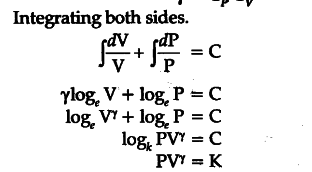# Derive the equation of adiabatic changes

(i) Consider 1 g mole of an ideal gas enclosed in a cylinder fitted with a perfectly friction less piston. Let P, V, T be initial pressure, volume and temperature.
Suppose a small amount of heat dQ spent in two ways:
Increasing the temperature, of gas by a small range
dT at constant volume = \${{C}_{v}}\$dT
(ii) Expansion of gas by a small volume dV portion of heat spent PdV